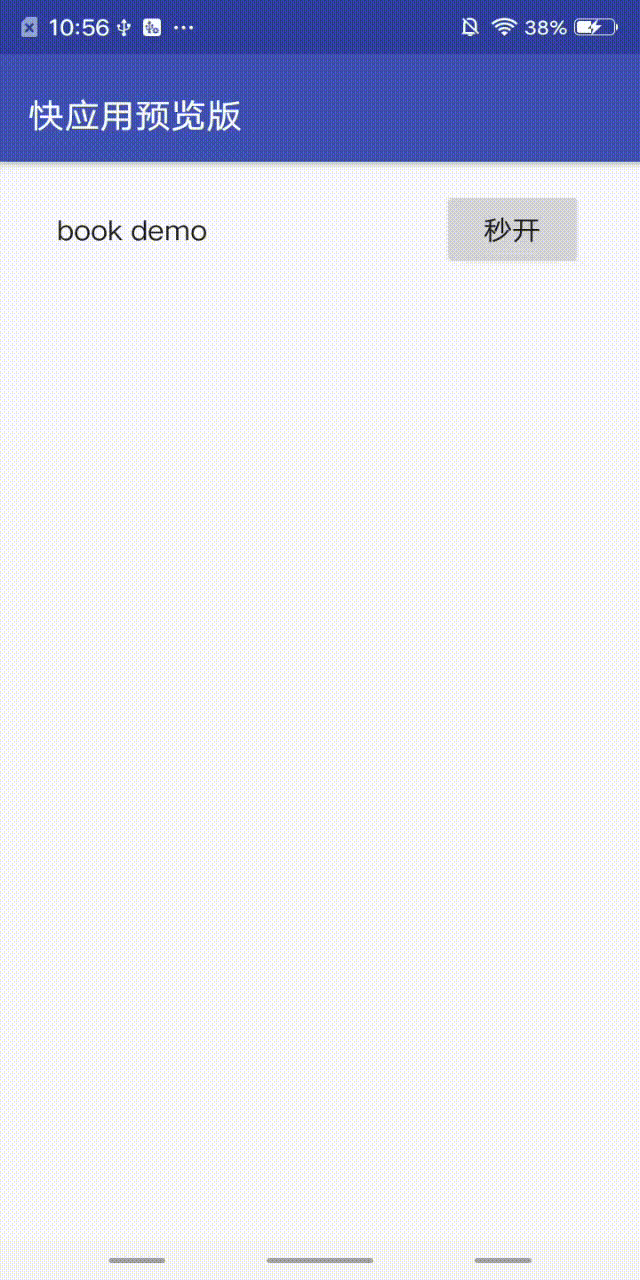# 骨架屏 `1090+`

## 配置方法

``````  src
|--- skeleton
|--- |--- page
|--- |    |--- page1.sk  骨架屏内容描述代码
|--- |    |--- page2.sk  骨架屏内容描述代码
|--- |--- config.json  配置页面和骨架屏的映射关系
``````

``````{
// 多对一配置："页面名称":"骨架屏内容描述文件"
"singleMap": {
"pages/main": "pages.main.sk",
"pages/sub": "pages.sub.sk",
"pages/other": "pages.sub.sk"
},

// 根据页面启动参数的一对多配置
"anchorMaps": [
{
"page": "pages/main" /* 页面名称 */,
"anchor": "paramsName" /* 所观察的启动参数名 */,
"skeletonMap": {
/* 参数值与对应的sk文件名映射表 */
"value1": "pages.main1.sk" /* 某个可能的参数值所对应的sk文件 */,
"value2": "pages.main2.sk",
"else": "pages.else.sk" /* 其他有效参数值所对应的sk文件 */,
"null": "pages.null.sk" /* 无该参数时所对应的sk文件 */
}
}
]
}
``````

``````<skeleton>
<clipPath width="800">
<rect x="40" y="40" width="720" height="300" rx="0" ry="0"/>
<rect x="40" y="410" width="720" height="45" rx="0" ry="0"/>
<rect x="40" y="517" width="720" height="60" rx="30" ry="30"/>
<rect x="62" y="527" width="15" height="40" rx="0" ry="0"/>
<rect x="120" y="527" width="60" height="40" rx="0" ry="0"/>
<rect x="670" y="527" width="60" height="40" rx="0" ry="0"/>
<rect x="40" y="617" width="720" height="60" rx="30" ry="30"/>
<rect x="61" y="627" width="17" height="40" rx="0" ry="0"/>
<rect x="120" y="627" width="60" height="40" rx="0" ry="0"/>
<rect x="670" y="627" width="60" height="40" rx="0" ry="0"/>
<rect x="40" y="717" width="720" height="60" rx="30" ry="30"/>
<rect x="61" y="727" width="17" height="40" rx="0" ry="0"/>
<rect x="120" y="727" width="90" height="40" rx="0" ry="0"/>
<rect x="670" y="727" width="60" height="40" rx="0" ry="0"/>
<rect x="40" y="827" width="720" height="45" rx="0" ry="0"/>
<rect x="40" y="933" width="180" height="240" rx="7" ry="7"/>
<rect x="250" y="933" width="106" height="45" rx="0" ry="0"/>
<rect x="250" y="989" width="51" height="33" rx="0" ry="0"/>
<rect x="250" y="1032" width="510" height="63" rx="0" ry="0"/>
<rect x="250" y="1106" width="81" height="33" rx="0" ry="0"/>
</clipPath>
</skeleton>
``````

`````` onShow() {
setTimeout(() => {
this.\$page.hideSkeleton()
}, 1500)
}
``````## sk 文件语法规范

`<skeleton>`根标签。仅包含 1 种子标签`<clipPath>`，但可以包含多个
`<clipPath>`描述一个骨架内容，仅包含 4 种子标签：`<rect>``<circle>``<ellipse>``<full-screen-img>`1. width：必选字段，骨架视图的宽度
2. height：可选字段，骨架视图的高度。当存在 footer 属性为 true 的子标签时，此字段必需填写
3. color：可选字段，整个骨架视图的背景颜色，默认为 manifest 中配置的窗口背景颜色
4. autoHide：可选字段，是否自动隐藏骨架屏，默认为 true。true：自动隐藏，时机是在页面创建完成时；false：开发者在页面生命周期内调用 this.\$page.hideSketelon()方法来主动隐藏骨架屏
5. media：基于媒体查询匹配骨架内容
`<rect>`矩形骨架元素1. width：必选字段，矩形宽度
2. height：必选字段，矩形高度
3. x：必选字段，距离骨架左侧边缘的距离
4. y：必选字段，距离骨架顶部的距离
5. rx：可选字段，水平方向圆角半径。如果 rx 和 ry 仅有一个设置了，则 rx 和 ry 都将被设置为该值；如果 rx 或 ry 有一个被设置成 0，相当于 rx = 0 且 ry = 0，圆角无效
6. ry：可选字段，垂直方向圆角半径。如果 rx 和 ry 仅有一个设置了，则 rx 和 ry 都将被设置为该值；如果 rx 或 ry 有一个被设置成 0，相当于 rx = 0 且 ry = 0，圆角无效
7. color：可选字段，元素颜色，默认值为#EFEFEF
8. footer：可选字段，默认值为 false。当设置为 true 时（要求`<clipPath>`标签的`height`属性不为空），该元素将始终保持距离屏幕底部的相对距离，而不会因屏幕适配导致位置上移或下沉， 常用于固定页面底部的 tab 标签骨架
`<circle>`圆形骨架元素1. cx：必选字段，圆心距离骨架左侧边缘的距离
2. cy：必选字段，圆心距离骨架顶部的距离
3. r：必选字段，半径值
4. color：可选字段，元素颜色，默认值为#EFEFEF
5. footer，同`<rect>`标签
`<ellipse>`椭圆骨架元素1. cx：必选字段，圆心距离骨架左侧边缘的距离
2. cy：必选字段，圆心距离骨架顶部的距离
3. rx：必选字段，圆的 x 半径
4. ry：必选字段，圆的 y 半径
5. color：可选字段，元素颜色，默认值为#EFEFEF
6. footer，同`<rect>`标签
`<full-screen-img>`全屏图片元素1. local-src：必选字段，本地图片路径，不建议使用体积过大的图片
2. fit-style：可选字段，图片填充方式，默认 none
• contain：保持宽高比，缩小或者放大，使得图片完全显示在显示边界内，居中显示
• cover：保持宽高比，缩小或者放大，使得两边都大于或等于显示边界，居中显示
• fill：不保存宽高比，填充满显示边界
• none：居中，无缩放
• scale-down：保持宽高比，缩小或保持不变，取 contain 和 none 中显示较小的一个，居中显示

### 基于媒体查询的多设备匹配

`<clipPath>`标签添加`media`属性值后，开发者可以根据各种设备特征和参数的值或者是否存在来决定最终渲染哪一个骨架内容。media 属性的值是媒体特性表达式，其规范与快应用的媒体查询一致。

``````<skeleton>
<!-- 竖屏应用的骨架视图 -->
<clipPath width="1080" height="2280" media="orientation: portrait">
<full-screen-img local-src="/Common/portrait.jpg"/>
</clipPath>
<!-- 横屏应用的骨架视图 -->
<clipPath width="2280" height="1080" media="orientation: landscape">
<full-screen-img local-src="/Common/landscape.jpg"/>
</clipPath>
</skeleton>
``````

## Tips

1 不建议将骨架屏和产品表现需求绑定在一起。骨架是一种优化措施，并不是在任何场景下都能显示出来，页面特别简单或是在性能特别好的手机上，白屏的时间特别短，骨架屏可能显示不出来。

2 .sk 文件中的所有数值类型的属性值都不带单位，表示相对大小。

3 控制`<full-screen-img>`所依赖的图片资源体积，建议 300kb 以内。

5 IDE 在`v3.7.0`版本提供了骨架屏生成工具，支持根据调试的真机画面`一键生成`骨架视图描述代码（.sk 文件）和配置文件，极大地提高开发效率。当自动生成的骨架效果不理想时，开发者可再做调整。